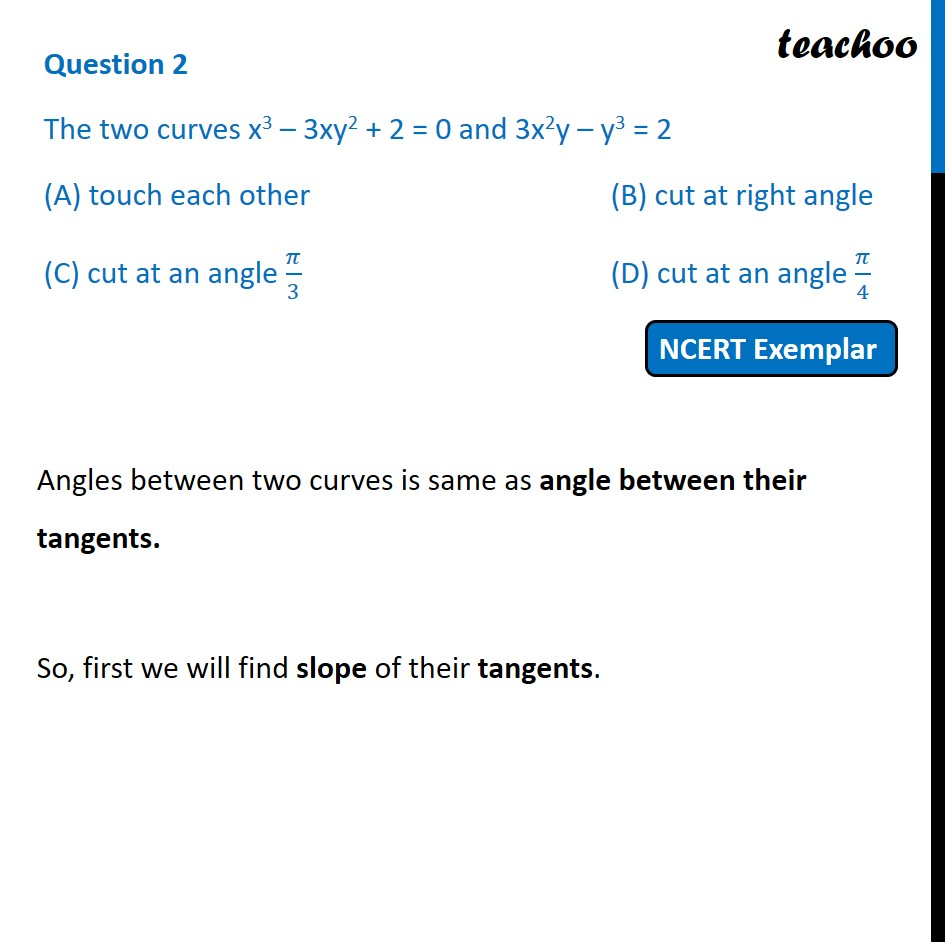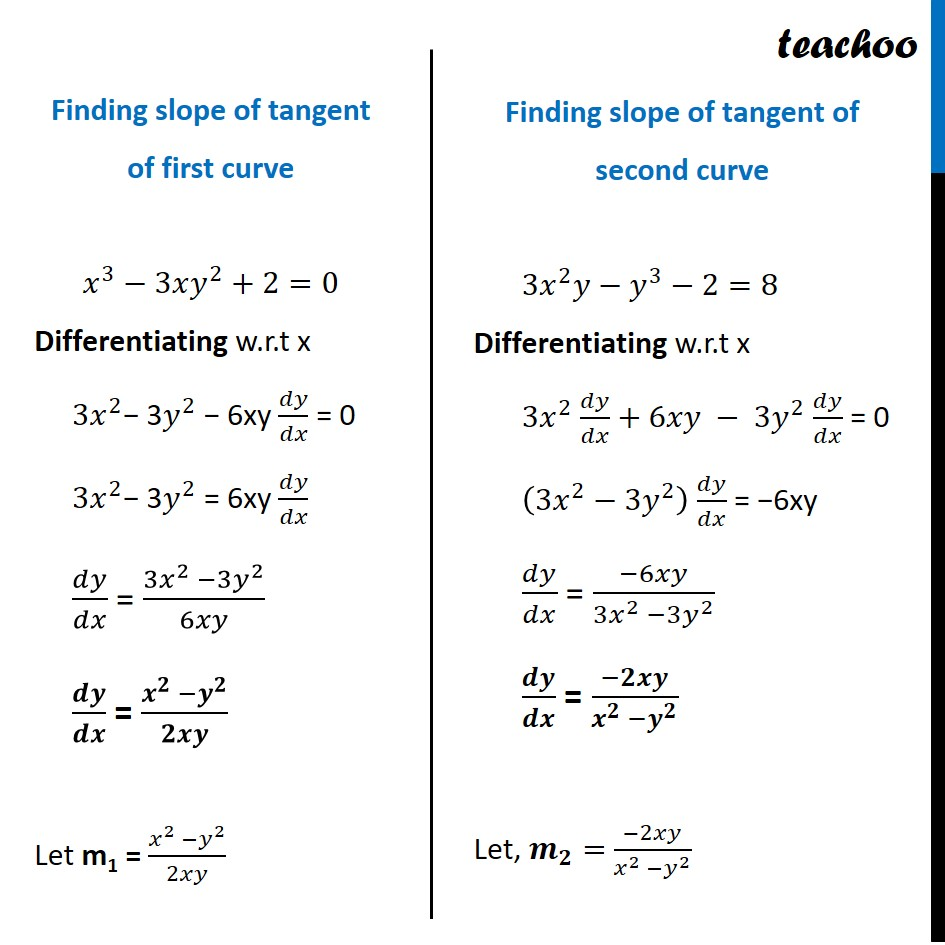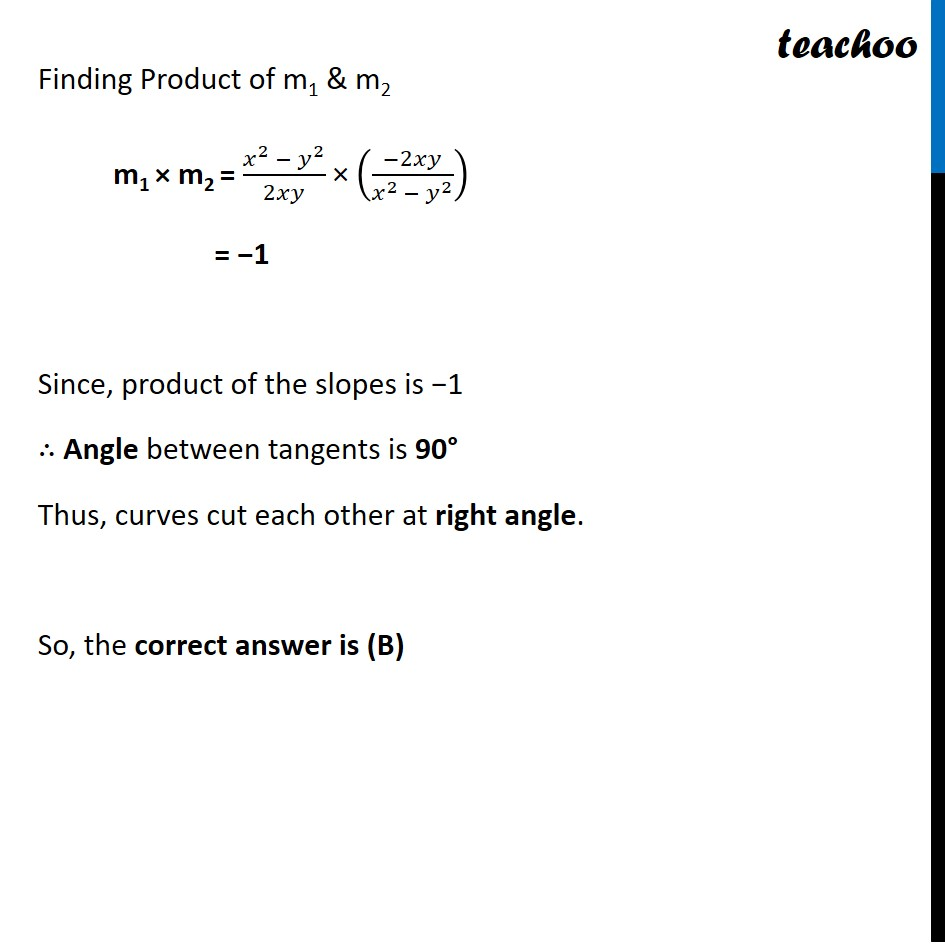NCERT Exemplar - MCQs

Chapter 6 Class 12 Application of Derivatives
Serial order wise

## (D) cut at an angle π/4Get live Maths 1-on-1 Classs - Class 6 to 12

### Transcript

Question 2 The two curves x3 – 3xy2 + 2 = 0 and 3x2y – y3 = 2 (A) touch each other (B) cut at right angle (C) cut at an angle 𝜋/3 (D) cut at an angle 𝜋/4 Angles between two curves is same as angle between their tangents. So, first we will find slope of their tangents. Finding slope of tangent of first curve 𝑥^3−3𝑥𝑦^2+2=0 Differentiating w.r.t x 〖3𝑥〗^2− 3𝑦^2 − 6xy 𝑑𝑦/𝑑𝑥 = 0 〖3𝑥〗^2− 3𝑦^2 = 6xy 𝑑𝑦/𝑑𝑥 𝑑𝑦/𝑑𝑥 = (3𝑥^2 −3𝑦^2)/6𝑥𝑦 𝒅𝒚/𝒅𝒙 = (𝒙^𝟐 −𝒚^𝟐)/𝟐𝒙𝒚 Let m1 = (𝑥^2 −𝑦^2)/2𝑥𝑦 Finding slope of tangent of second curve 3𝑥^2 𝑦−𝑦^3−2=8 Differentiating w.r.t x 3𝑥^2 𝑑𝑦/𝑑𝑥+6𝑥𝑦 − 3𝑦^2 𝑑𝑦/𝑑𝑥 = 0 (3𝑥^2−3𝑦^2 ) 𝑑𝑦/𝑑𝑥 = −6xy 𝑑𝑦/𝑑𝑥 = (−6𝑥𝑦)/(3𝑥^2 −3𝑦^2 ) 𝒅𝒚/𝒅𝒙 = (−𝟐𝒙𝒚)/(𝒙^𝟐 −𝒚^𝟐 ) Let, 𝒎_𝟐= (−2𝑥𝑦)/(𝑥^2 −𝑦^2 ) Finding Product of m1 & m2 m1 × m2 = (𝑥^2 − 𝑦^2)/2𝑥𝑦 × ((−2𝑥𝑦)/(𝑥^(2 )− 𝑦^2 )) = −1 Since, product of the slopes is −1 ∴ Angle between tangents is 90° Thus, curves cut each other at right angle. So, the correct answer is (B)